comm.SymbolSynchronizer

Correct symbol timing clock skew

Description

The comm.SymbolSynchronizer System object™ corrects symbol timing clock skew between a single-carrier transmitter and receiver for PAM, PSK, QAM, and OQPSK modulation schemes. For more information, see Symbol Synchronization Overview.

Note

The input signal operates on a sample-rate basis and the output signal operates on a symbol-rate basis.

To correct symbol timing clock skew:

1. Create the comm.SymbolSynchronizer object and set its properties.

2. Call the object with arguments, as if it were a function.

Creation

Description

example

symbolSync = comm.SymbolSynchronizer creates a symbol synchronizer System object for correcting the clock skew between a single-carrier transmitter and receiver.

example

symbolSync = comm.SymbolSynchronizer(Name,Value) sets properties using one or more name-value pairs. For example, comm.SymbolSynchronizer('Modulation','OQPSK') configures the symbol synchronizer System object for an OQPSK-modulated input signal. Enclose each property name in quotes.

Tunable DampingFactor, NormalizedLoopBandwidth, and DetectorGain properties enable you to optimize synchronizer performance in your simulation loop without releasing the object.

Properties

expand all

Unless otherwise indicated, properties are nontunable, which means you cannot change their values after calling the object. Objects lock when you call them, and the release function unlocks them.

If a property is tunable, you can change its value at any time.

Modulation type, specified as 'PAM/PSK/QAM' or 'OQPSK'.

Tunable: No

Data Types: char | string

Timing error detector method, specified as Zero-Crossing (decision-directed), Gardner (non-data-aided), Early-Late (non-data-aided), or Mueller-Muller (decision-directed). This property assigns the timing error detection scheme used in the synchronizer. For more information, see Timing Error Detection (TED).

Tunable: No

Data Types: char | string

Samples per symbol, specified as an integer greater than 1.

Tunable: No

Data Types: double

Damping factor of the loop filter, specified as a positive scalar. For more information, see Loop Filter.

Tunable: Yes

Data Types: double | single

Normalized bandwidth of the loop filter, specified as a scalar in the range (0, 1). The loop bandwidth is normalized to the sample rate of the input signal. For more information, see Loop Filter.

Note

To ensure the symbol synchronizer locks, set the NormalizedLoopBandwidth property to a value less than 0.1.

Tunable: Yes

Data Types: double | single

Phase detector gain, specified as a positive scalar.

Tunable: Yes

Data Types: double | single

Usage

For versions earlier than R2016b, use the step function to run the System object algorithm. The arguments to step are the object you created, followed by the arguments shown in this section.

For example, y = step(obj,x) and y = obj(x) perform equivalent operations.

Description

symbols = symbolSync(samples) corrects symbol timing clock skew between a single-carrier transmitter and receiver based on the input samples and outputs synchronized symbols.

• The input operates on a sample-rate basis and the output signal operates on a symbol-rate basis.

• You can tune the DampingFactor, NormalizedLoopBandwidth, and DetectorGain properties to improve the synchronizer performance.

Input Arguments

expand all

Input samples, specified as a scalar or column vector of a PAM-, PSK-, QAM-, or OQPSK-modulated single-carrier signal.

Data Types: double | single
Complex Number Support: Yes

Output Arguments

expand all

Synchronized symbols, returned as a variable-sized column vector. The output symbols inherit the data type from the input samples. For an input with dimensions Nsamp-by-1, this output has dimensions Nsym-by-1. Nsym is approximately equal to Nsamp divided by Nsps, where Nsps is equal to the SamplesPerSymbol property value. If the output exceeds the maximum output size of $⌈\frac{{N}_{\text{samp}}}{{N}_{\text{sps}}}×1.1⌉$, it is truncated.

Estimated timing error for each input sample, returned as a scalar in the range [0, 1] or column vector of elements in the range [0, 1]. The estimated timing error is normalized to the input sample rate. timingErr has the same data type and size as input samples.

Object Functions

To use an object function, specify the System object as the first input argument. For example, to release system resources of a System object named obj, use this syntax:

release(obj)

expand all

 clone Create duplicate System object isLocked Determine if System object is in use
 step Run System object algorithm release Release resources and allow changes to System object property values and input characteristics reset Reset internal states of System object

Examples

collapse all

Correct a fixed symbol timing error on a noisy QPSK-modulated signal. Check the bit error rate (BER) of the synchronized received signal.

Initialize simulation parameters.

M = 4;         % Modulation order for QPSK
nSym = 5000;   % Number of symbols in a packet
sps = 4;       % Samples per symbol
timingErr = 2; % Samples of timing error
snr = 15;      % Signal-to-noise ratio (dB)

Create root raised cosine (RRC) transmit and receive filter System objects.

txfilter = comm.RaisedCosineTransmitFilter( ...
'OutputSamplesPerSymbol',sps);
'InputSamplesPerSymbol',sps,'DecimationFactor',2);

Create a symbol synchronizer System object to correct the timing error.

symbolSync = comm.SymbolSynchronizer;

Generate random M-ary symbols and apply QPSK modulation.

data = randi([0 M-1],nSym,1);
modSig = pskmod(data,M,pi/4);

Create a delay object to introduce a fixed timing error of 2 samples. Because the transmit RRC filter outputs 4 samples per symbol, 1 sample is equivalent to a 1/4 symbol through the fixed delay and channel.

fixedDelay = dsp.Delay(timingErr);
fixedDelaySym = ceil(fixedDelay.Length/sps); % Round fixed delay to nearest integer in symbols

Filter the modulated signal through a transmit RRC filter by using the txfilter object. Apply a signal timing error by using the fixedDelay object.

txSig = txfilter(modSig);
delaySig = fixedDelay(txSig);

Pass the delayed signal through an AWGN channel with a 15 dB signal-to-noise ratio.

rxSig = awgn(delaySig,snr,'measured');

Filter the modulated signal through a receive RRC filter by using the rxfilter object. Display the scatter plot. Due to the timing error, the received signal does not align with the expected QPSK reference constellation.

rxSample = rxfilter(rxSig);
scatterplot(rxSample(1001:end),2)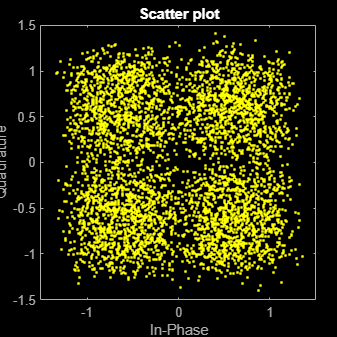Correct the symbol timing error by using the symbolSync object. Display the scatter plot. The synchronized signal now aligns with the expected QPSK constellation.

rxSync = symbolSync(rxSample);
scatterplot(rxSync(1001:end),2)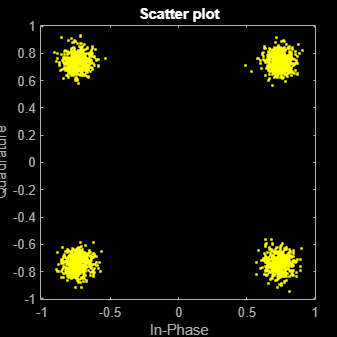Demodulate the QPSK signal.

recData = pskdemod(rxSync,M,pi/4);

Compute, in symbols, the total system delay due to the fixed delay and the transmit and receive RRC filters.

sysDelay = dsp.Delay(fixedDelaySym + txfilter.FilterSpanInSymbols/2 + ...
rxfilter.FilterSpanInSymbols/2);

Compute the BER, taking into account the system delay.

[numErr,ber] = biterr(sysDelay(data),recData)
numErr = 12
ber = 0.0012

Correct a fixed symbol timing error on a noisy BPSK transmission signal. Check the bit error rate (BER) of the synchronized received signal.

Initialize simulation parameters.

M = 2;         % Modulation order for BPSK
nSym = 20000;  % Number of symbols in a packet
sps = 4;       % Samples per symbol
timingErr = 2; % Samples of timing error
snr = 15;      % Signal-to-noise ratio (dB)

Create root raised cosine (RRC) transmit and receive filter System objects.

txfilter = comm.RaisedCosineTransmitFilter(...
'OutputSamplesPerSymbol',sps);
'InputSamplesPerSymbol',sps,'DecimationFactor',1);

Create a symbol synchronizer System object™ to correct the timing error.

symbolSync = comm.SymbolSynchronizer(...
'SamplesPerSymbol',sps, ...
'NormalizedLoopBandwidth',0.01, ...
'DampingFactor',1.0, ...
'TimingErrorDetector','Early-Late (non-data-aided)');

Generate random data symbols and apply BPSK modulation.

data = randi([0 M-1],nSym,1);
modSig = pskmod(data,M);

Create a delay object to introduce a fixed timing error of 2 samples. Because the transmit RRC filter outputs 4 samples per symbol, 1 sample is equivalent to a 1/4 symbol through the fixed delay and channel.

fixedDelay = dsp.Delay(timingErr);
fixedDelaySym = ceil(fixedDelay.Length/sps); % Round fixed delay to nearest integer in symbols

Filter the modulated signal through a transmit RRC filter by using the txfilter object. Apply a signal timing error by using the fixedDelay object.

txSig = txfilter(modSig);
delayedSig = fixedDelay(txSig);

Pass the delayed signal through an AWGN channel.

rxSig = awgn(delayedSig,snr,'measured');

Filter the modulated signal through a receive RRC filter by using the rxfilter object. Display the scatter plot. Due to the timing error, the received signal does not align with the expected BPSK reference constellation.

rxSample = rxfilter(rxSig);
scatterplot(rxSample(10000:end),2)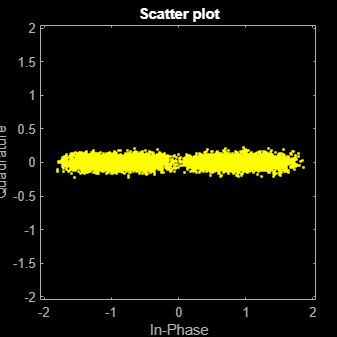Correct the symbol timing error by using the symbolSync object. Display the scatter plot. The synchronized signal now aligns with the expected BPSK constellation.

rxSync = symbolSync(rxSample);
scatterplot(rxSync(10000:end),2)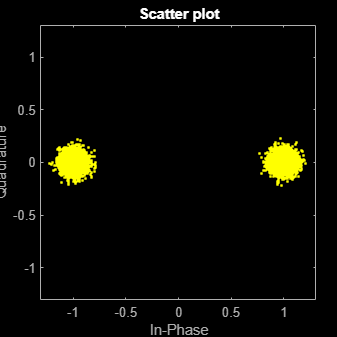Demodulate the BPSK signal.

recData = pskdemod(rxSync,M);

Compute, in symbols, the total system delay due to the fixed delay and the transmit and receive RRC filters.

sysDelay = dsp.Delay(fixedDelaySym + txfilter.FilterSpanInSymbols/2 + ...
rxfilter.FilterSpanInSymbols/2);

Compute the BER, taking into account the system delay.

[numErr1,ber1] = biterr(sysDelay(data),recData)
numErr1 = 8
ber1 = 4.0000e-04

Correct symbol timing and frequency offset errors by using the comm.SymbolSynchronizer and comm.CarrierSynchronizer System objects.

Configuration

Initialize simulation parameters.

M = 16;           % Modulation order
nSym = 2000;      % Number of symbols in a packet
sps = 2;          % Samples per symbol
spsFilt = 8;      % Samples per symbol for filters and channel
spsSync = 2;      % Samples per symbol for synchronizers
lenFilt = 10;     % RRC filter length

Create a matched pair of root raised cosine (RRC) filter System objects for transmitter and receiver.

txfilter = comm.RaisedCosineTransmitFilter('FilterSpanInSymbols',lenFilt, ...
'OutputSamplesPerSymbol',spsFilt,'Gain',sqrt(spsFilt));
'InputSamplesPerSymbol',spsFilt,'DecimationFactor',spsFilt/2,'Gain',sqrt(1/spsFilt));

Create a phase-frequency offset System object to introduce a 100 Hz Doppler shift.

doppler = comm.PhaseFrequencyOffset('FrequencyOffset',100, ...
'PhaseOffset',45,'SampleRate',1e6);

Create a variable delay System object to introduce timing offsets.

varDelay = dsp.VariableFractionalDelay;

Create carrier and symbol synchronizer System objects to correct for Doppler shift and timing offset, respectively.

carrierSync = comm.CarrierSynchronizer('SamplesPerSymbol',spsSync);
symbolSync = comm.SymbolSynchronizer( ...
'TimingErrorDetector','Early-Late (non-data-aided)', ...
'SamplesPerSymbol',spsSync);

Create constellation diagram System objects to view the results.

refConst = qammod(0:M-1,M,'UnitAveragePower',true);
cdDoppler = comm.ConstellationDiagram('ReferenceConstellation',refConst, ...
'SamplesPerSymbol',spsSync,'Title','Frequency Corrected Signal');
cdTiming = comm.ConstellationDiagram('ReferenceConstellation',refConst, ...
'SamplesPerSymbol',spsSync,'Title','Frequency and Timing Synchronized Signal');

Main Processing Loop

The main processing loop:

• Generates random symbols and apply QAM modulation.

• Filters the modulated signal.

• Applies frequency and timing offsets.

• Passes the transmitted signal through an AWGN channel.

• Corrects the Doppler shift.

• Corrects the timing offset.

for k = 1:15
data = randi([0 M-1],nSym,1);
modSig = qammod(data,M,'UnitAveragePower',true);
txSig = txfilter(modSig);

txDoppler = doppler(txSig);
txDelay = varDelay(txDoppler,k/15);

rxSig = awgn(txDelay,25);

rxFiltSig = rxfilter(rxSig);
rxCorr = carrierSync(rxFiltSig);
rxData = symbolSync(rxCorr);
end

Visualization

Plot the constellation diagrams of the received signal, frequency corrected signal, and frequency and timing synchronized signal. Specific constellation points cannot be identified in the received signal and can be only partially identified in the frequency corrected signal. However, the timing and frequency synchronized signal aligns with the expected QAM constellation points.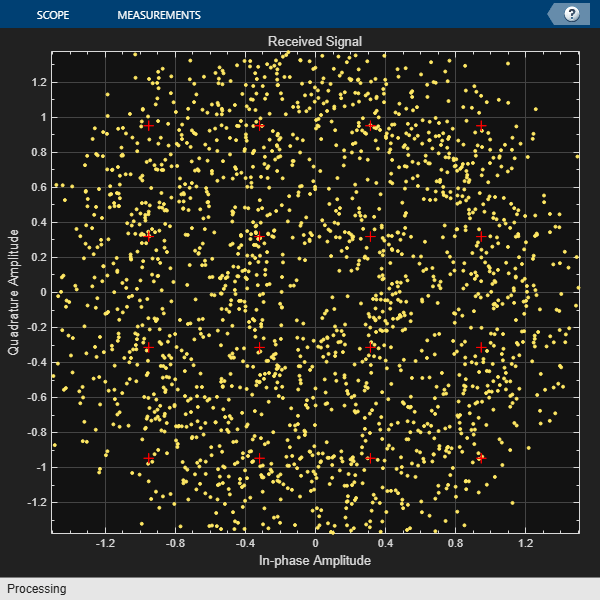cdDoppler(rxCorr)cdTiming(rxData)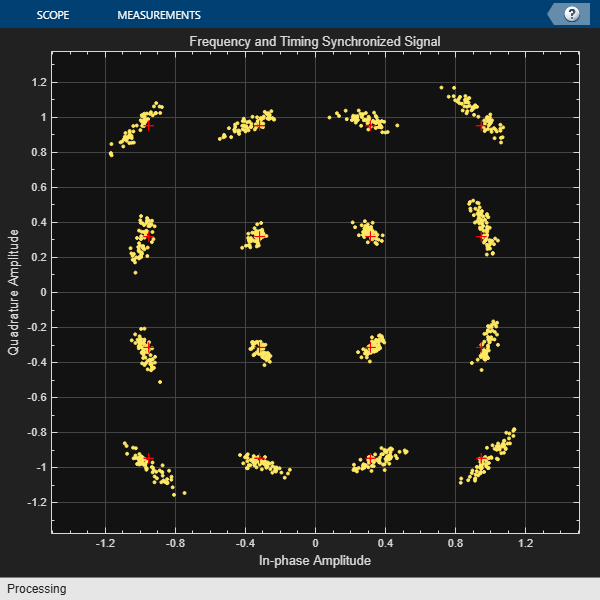Correct a monotonically increasing symbol timing error on a noisy 8-PSK signal. Display the normalized timing error.

Initialize simulation parameters.

M = 8;            % Modulation order
nSym = 5000;      % Number of symbol in a packet
sps = 2;          % Samples per symbol
nSamp = sps*nSym; % Number of samples in a packet

Create root raised cosine (RRC) transmit and receive filter System objects.

txfilter = comm.RaisedCosineTransmitFilter( ...
'OutputSamplesPerSymbol',sps);
'InputSamplesPerSymbol',sps, ...
'DecimationFactor',1);

Create a variable fractional delay System object™ to introduce a monotonically increasing timing error.

varDelay = dsp.VariableFractionalDelay;

Create a symbol synchronizer System object to correct the timing error.

symbolSync = comm.SymbolSynchronizer(...
'TimingErrorDetector','Mueller-Muller (decision-directed)', ...
'SamplesPerSymbol',sps);

Generate random 8-ary symbols and apply 8-PSK modulation.

data = randi([0 M-1],nSym,1);
modSig = pskmod(data,M,pi/8);

Filter the modulated signal through a raised cosine transmit filter and apply a monotonically increasing timing delay.

vdelay = (0:1/nSamp:1-1/nSamp)';
txSig = txfilter(modSig);
delaySig = varDelay(txSig,vdelay);

Pass the delayed signal through an AWGN channel with a 15 dB signal-to-noise ratio.

rxSig = awgn(delaySig,15,'measured');

Filter the modulated signal through a receive RRC filter. Display the scatter plot. Due to the timing error, the received signal does not align with the expected 8-PSK reference constellation.

rxSample = rxfilter(rxSig);
scatterplot(rxSample,sps)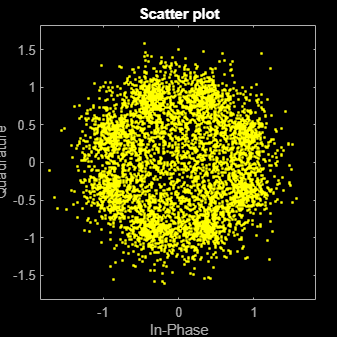Correct the symbol timing error by using the symbolSync object. Display the scatter plot. The synchronized signal now aligns with the expected 8-PSK constellation.

[rxSym,tError] = symbolSync(rxSample);
scatterplot(rxSym(1001:end))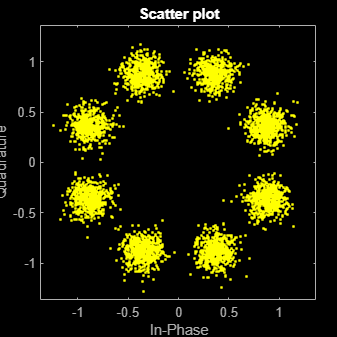Plot the timing error estimate. Over time, the normalized timing error increases to 1 sample.

figure
plot(vdelay,tError)
xlabel('Time (s)')
ylabel('Timing Error (samples)')